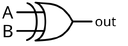## FANDOM

67 Pages

 INPUTA   B OUTPUTA XOR B 0 0 0 0 1 1 1 0 1 1 1 0

The XOR gate is a digital logic gate that implements exclusive disjunction - it behaves according to the truth table to the right. A HIGH output (1) results if one, and only one, of the inputs to the gate is HIGH (1). If both inputs are LOW (0) or both are HIGH (1), a LOW output (0) results.

This function is addition modulo 2. As a result, XOR gates are used to implement binary addition in computers. A half adder consists of an XOR gate and an AND gate.

## SymbolsEdit

There are two symbols for XOR gates: the 'military' symbol and the 'rectangular' symbol. For more information see Logic Gate Symbols'Military' XOR Symbol'Rectangular' XOR Symbol

Hardware description and pinout ==

XOR gates are basic logic gates, and as such they are recognised in TTL and CMOS ICs. The standard, 4000 series, CMOS IC is the 4070, which includes four independent, two-input, XOR gates. The 4070 replaces the less reliable 4030, but keeps the pinout. The pinout diagram is as follows:

 ```1 Input A1 2 Input B1 3 Output Q1 4 Input A2 5 Input B2 6 Output Q2 7 VG 8 Input A3 9 Input B3 10 Output Q3 11 Input A4 12 Input B4 13 Output Q4 14 VCC ```

This device is available from most semiconductor manufacturers such as Philips. It is usually available in both through-hole DIL and SOIC format. Datasheets are readily available in most Datasheet Databases.

## AlternativesEdit

If no specific XOR gates are available, one can be made from four NAND or five NOR gates in the configurations shown below. Interestingly, any logic gate can be made from a combination of NAND gates or a combination of NOR gates.

## More than two inputsEdit

Although generation of wider gates by simply adding additional inputs is valid for AND/NAND and OR/NOR, this is not valid for XOR.

Adding additional inputs to an XOR gate, while leaving the =1 notation, defines a gate whose output is true if and only if exactly one of its inputs is true. For more than two inputs, this definition does not match common electronics industry usage of the term XOR.

In common usage, an XOR is used to mean that the output is true when an odd number of its inputs is true (false otherwise). This definition produces the a truth table for all values of n (including 2, and indeed, for n=1 and n=0), and when multiple two-input XOR's are cascaded, the same logic function results.